## Indices log extra note

Indices$\text{Positive Index: }{{a}^{n}},a\ne 0$$eg:{{2}^{3}},{{4}^{2}}$$\text{Negative Index: }{{a}^{-n}}=\frac{1}{{{a}^{n}}}$$eg:{{2}^{-3}}=\frac{1}{{{2}^{3}}}=\frac{1}{8}$$\text{Zero Index: }{{a}^{0}}=1,a\ne 0$$eg:{{2}^{0}}=1$

Fractional Indices:${{a}^{\frac{1}{n}}}=\sqrt[n]{a},a\ne 0$$eg:{{8}^{\frac{1}{3}}}=\sqrt{8}=2$${{a}^{\frac{m}{n}}}={{(\sqrt[n]{a})}^{m}},a\ne 0$$eg:{{8}^{\frac{2}{3}}}={{(\sqrt{8})}^{2}}={{(2)}^{2}}=4$

Law of Indices:$\text{Rule 1: }{{a}^{m}}\times {{a}^{n}}={{a}^{m+n}}$$eg:{{2}^{2}}\times {{2}^{3}}={{2}^{2+3}}={{2}^{5}}=32$$\text{Rule 2: }{{a}^{m}}\div {{a}^{n}}={{a}^{m-n}}$$eg:{{2}^{4}}\times {{2}^{2}}={{2}^{4-2}}={{2}^{2}}=4$$\text{Rule 3: }{{({{a}^{m}})}^{n}}={{a}^{m\times n}}$$eg:{{({{2}^{2}})}^{3}}={{2}^{2\times 3}}={{2}^{6}}=64$

How to simplify algebraic expressions

Example 1:$\begin{array}{rcl} {{2}^{n+4}}-{{2}^{n-1}} &=&{{2}^{n}}\times {{2}^{4}}-{{2}^{n}}\times {{2}^{-1}} \text{[Split the power, use Rule 1]}\\ &=&{{2}^{n}}({{2}^{4}}-{{2}^{-1}}) \text{ [Factorize }{{\text{2}}^{n}}]\\ &=&{{2}^{n}}(16-\frac{1}{2}) \text{ [Simplify the fraction]}\\ &=&{{2}^{n}}(\frac{31}{2})\\ &=&{{2}^{n}}(31\times {{2}^{-1}}) \text{[Convert }\frac{1}{2}\text{into index form of }{{\text{2}}^{-1}}\text{] }\\ &=&31({{2}^{n-1}})\text{[Join }{{\text{2}}^{n}}\times \text{ }{{\text{2}}^{-1}}\text{ by using Rule 1]} \end{array}$

Example 2:$\begin{array}{rcl} {{4}^{3n}}\times {{4}^{-1}}\div {{4}^{3}} &=&{{4}^{3n+(-1)-3}}\text{ }\left[ \text{Join the power together, use Rule 1 , 2} \right] \\ &=&{{4}^{3n-4}} \end{array}$

Example 3:$\begin{array}{rcl} & {{4}^{2n-1}}\times {{2}^{n+3}} \\ &=&{{({{2}^{2}})}^{(2n-1)}}\times {{2}^{n+3}}\text{ }[\text{Change base 4 to base 2},\text{ 4}={{2}^{2}}] \\ &=&{{2}^{4n-2}}\times {{2}^{n+3}}\text{ }\!\![\!\!\text{ Use Rule 3, }{{({{2}^{2}})}^{(2n-1)}}={{2}^{4n-2}}] \\ &=&{{2}^{4n-2+n+3}}\text{ }[\text{Join the power, use Rule 1}] \\ &=&{{2}^{5n+1}} \\ \end{array}$

Logarithms

If$N={{a}^{x}}$ then${{\log }_{a}}N=x$. So$N={{a}^{x}}\leftrightarrow {{\log }_{a}}N=x$. For example, if$16={{2}^{4}}$, then${{\log }_{2}}16=4$, where index 4 becomes the logarithms and 2 as the base.

In general,${{\log }_{10}}x=\log x=\lg x$ , we call them as common logarithms (base 10). The [log] where you can find from calculator is the common logarithm.

Example 4:

Find the value of${{\log }_{10}}19$

Example 5:

Solve${{\log }_{10}}m=-2.5686$

Answer: m = 0.0027 [Press (shift)(log)(-2.5686)]

Laws of Logarithms:

1)${{\log }_{a}}xy={{\log }_{a}}x+{{\log }_{a}}y$

Example 6:${{\log }_{6}}54+{{\log }_{6}}4$$\begin{array}{rcl} & =&{{\log }_{6}}54\times 4\left[ \text{Join the logarithm} \right ] \\ & =&{{\log }_{6}}216 \\ & =&{{\log }_{6}}{{6}^{3}}\text{ }\!\! [ \!\!\text{ }216={{6}^{3}}] \\ & =&3{{\log }_{6}}6\left[ \text{bring the power to the front} \right ] \\ & =&3(1)\text{ }\!\![\!\!\text{ }{{\log }_{n}}n=1,\text{ so }{{\log }_{6}}6=1\text{ }\!\!]\!\!\text{ } \\ & =&3 \\ \end{array}$

2)${{\log }_{a}}\frac{x}{y}={{\log }_{a}}x-{{\log }_{a}}y$

Example 7:${{\log }_{3}}54-{{\log }_{3}}2$$\begin{array}{rcl} & =&{{\log }_{3}}\frac{54}{2} \\ & =&{{\log }_{3}}27 \\ & =&{{\log }_{3}}{{3}^{3}} \\ & =&3{{\log }_{3}}3 \\ & =&3(1) \\ & =&3 \\ \end{array}$

3)${{\log }_{a}}{{x}^{q}}=q{{\log }_{a}}x$

Example 8:${{\log }_{5}}125$$\begin{array}{rcl} & =&{{\log }_{5}}{{5}^{3}} \\ & =&3{{\log }_{5}}5 \\ & =&3(1) \\ & =&3 \\ \end{array}$

4)${{\log }_{a}}1=0$

Example 9:${{\log }_{100}}1=0$

5)${{\log }_{a}}a=1$

Example 10:${{\log }_{2}}2=1$ ,${{\log }_{100}}100=1$

Change the Base of Logarithm

1)${{\log }_{a}}b=\frac{{{\log }_{c}}b}{{{\log }_{c}}a}$

2)${{\log }_{a}}b=\frac{1}{{{\log }_{b}}a}$

Example 11:

Evaluate${{\log }_{5}}8$${{\log }_{5}}8$$=\frac{{{\log }_{10}}8}{{{\log }_{10}}5}$$=1.292$

The following examples need to be solved using the Laws of Logarithms and change of base. So please remember the laws of logarithms and the change of the base of logarithms.

Example 12:

Find the value of${{\log }_{\sqrt{3}}}8\times {{\log }_{2}}7\times {{\log }_{7}}3$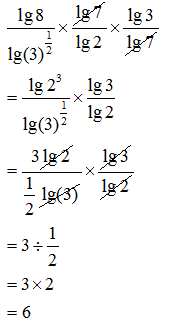Example 13:

Simplify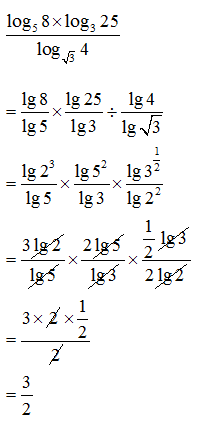Solving Equation involving indices and logarithms

a) Method 1: Expressing the equation to same base and compare the indices.

a) Method 1: Expressing the equation to same base and compare the indices.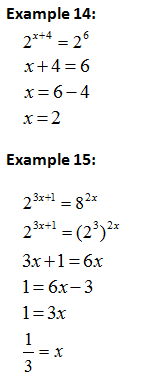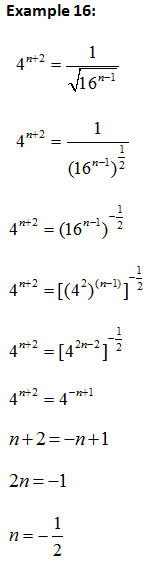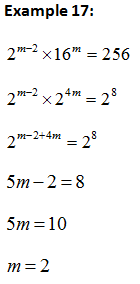b) Method 2: Expressing the equation to same indices and compare the base.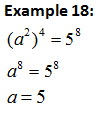c) Method 3: Using$y={{a}^{x}}$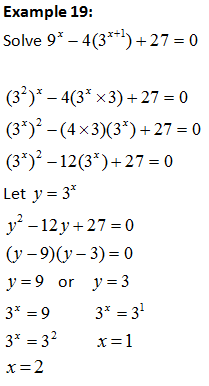d) Method 4: Expressing the equation as a single logarithms form to the same base